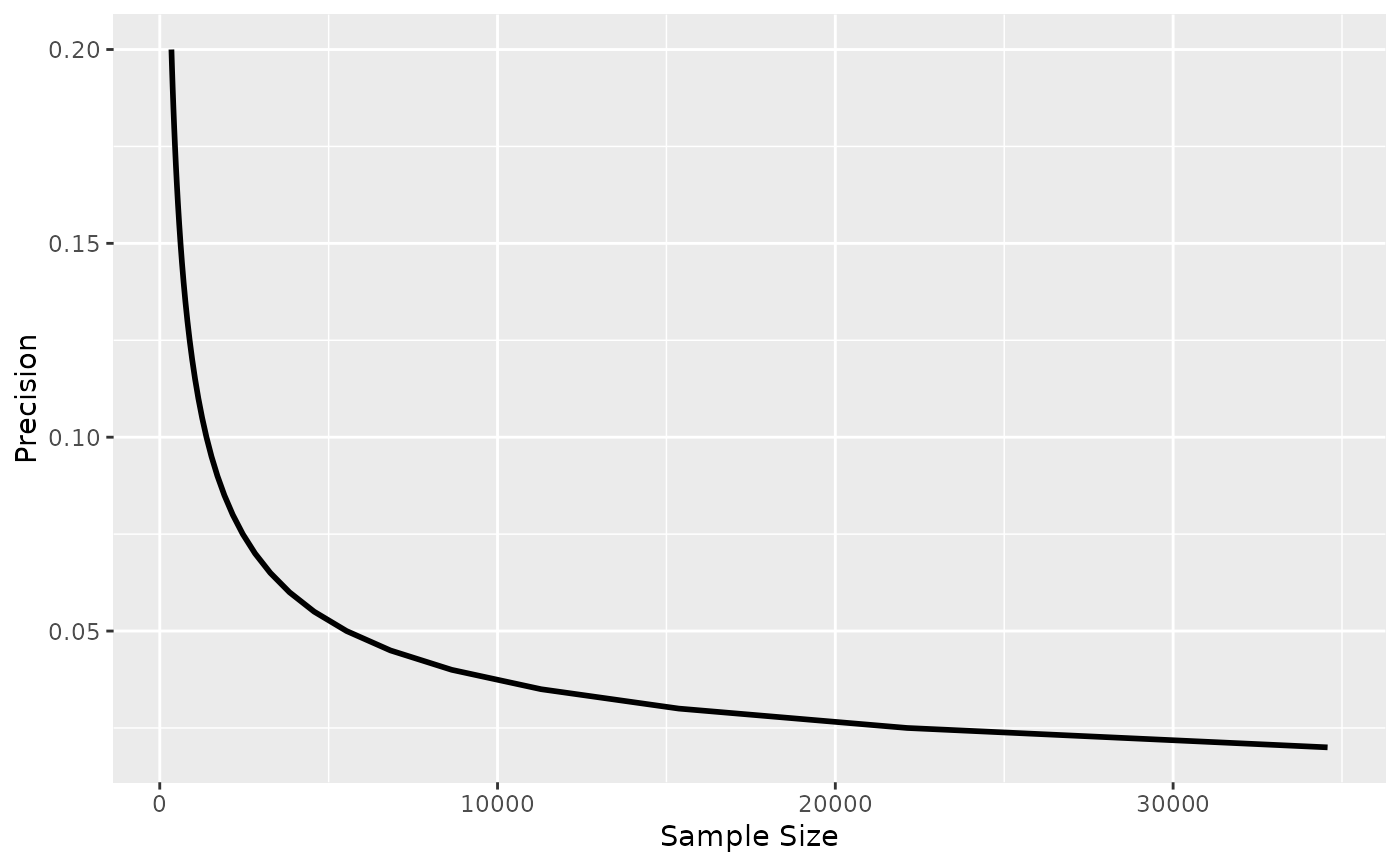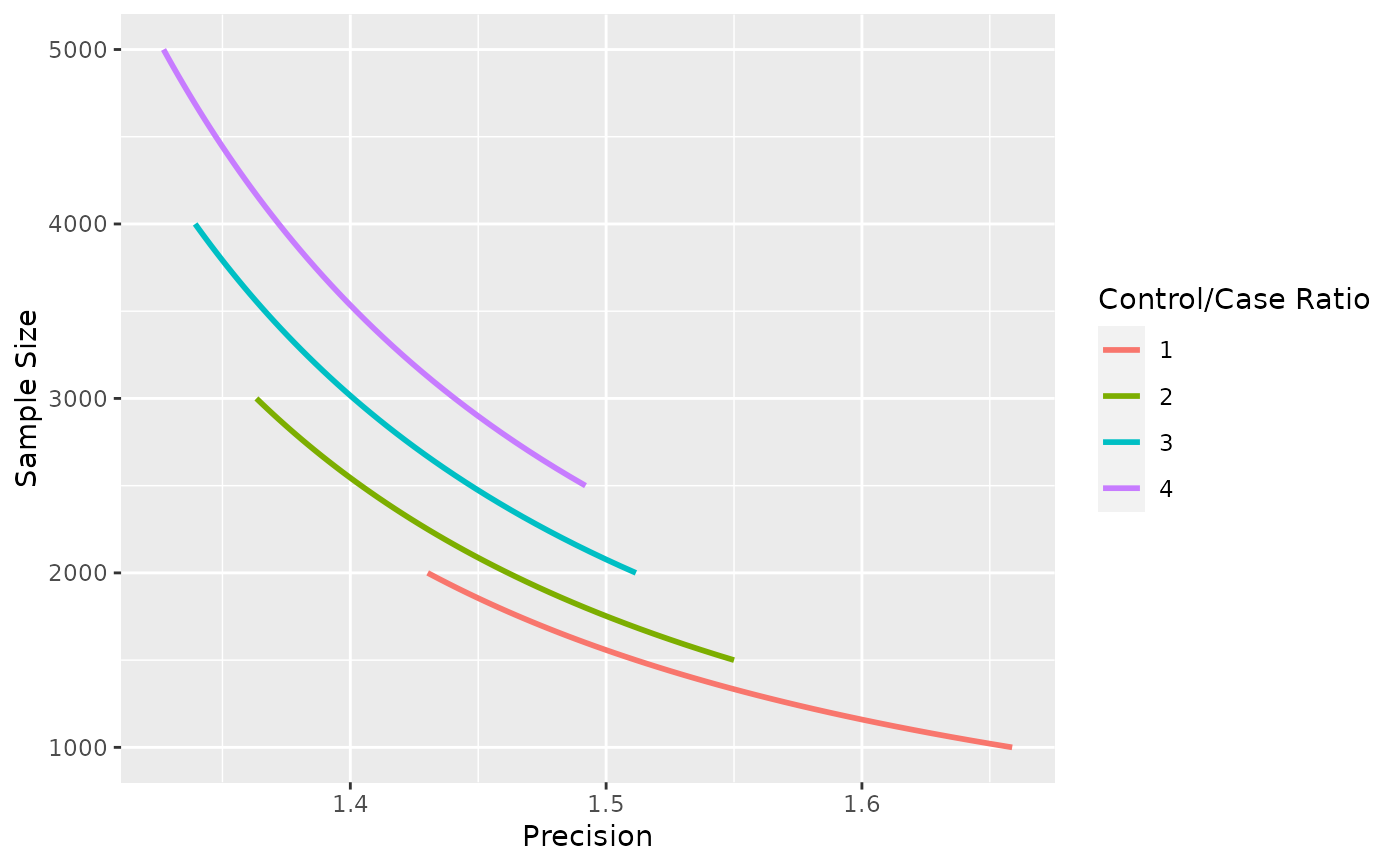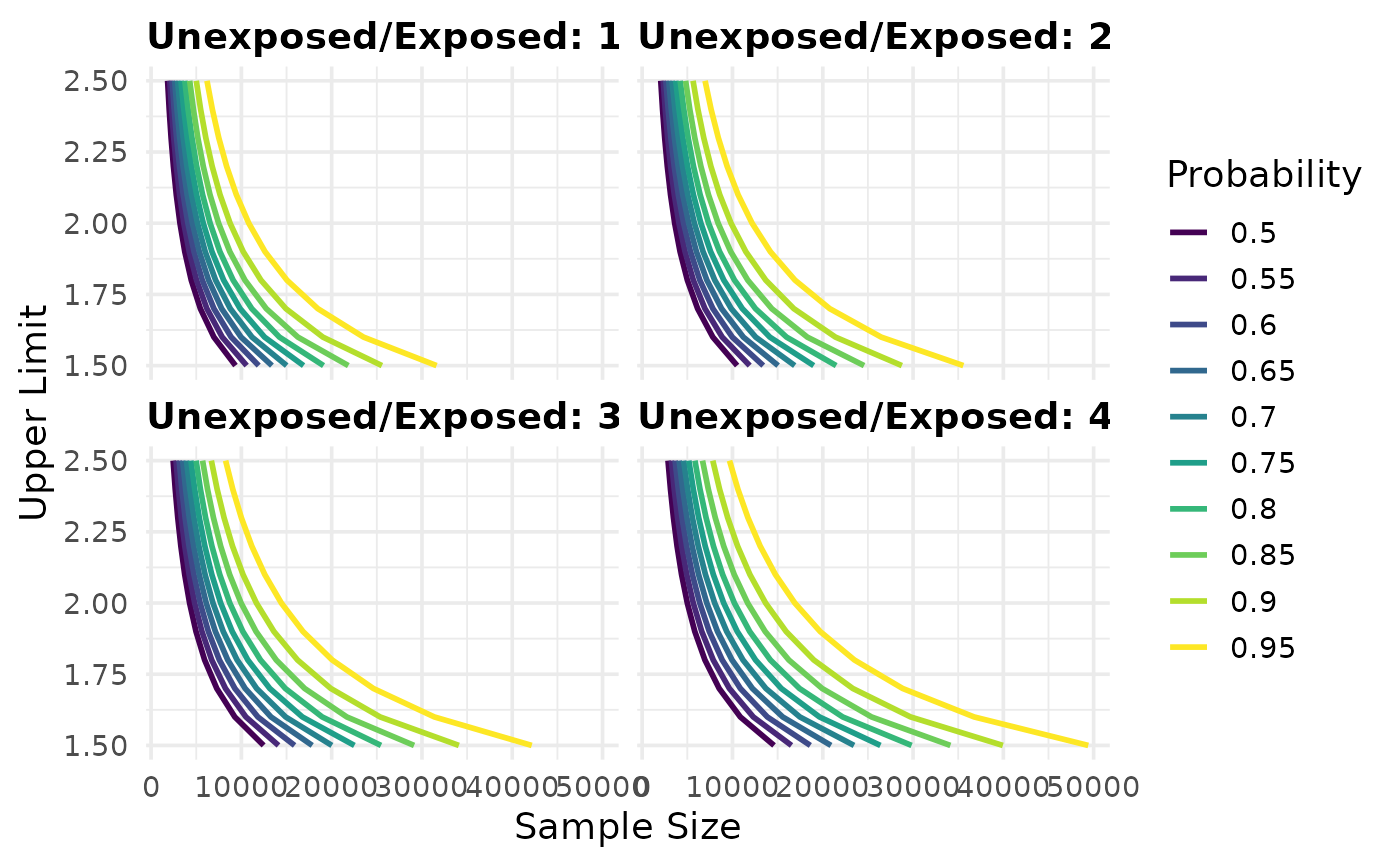Simple line plots for the output of map_precisely(). Use dplyr::group_by() to create multiple lines on the plot.

plot_sample_size(.df, xlab = "Sample Size", ylab = "Precision", line_size = 1)

plot_precision(.df, xlab = "Precision", ylab = "Sample Size", line_size = 1)

plot_upper_limit(
.df,
xlab = "Sample Size",
ylab = "Upper Limit",
line_size = 1
)

## Arguments

.df a data frame with values to plot, possibly from map_precisely(). Label for the x-axis. Label for the y-axis. The width of the line. Default is 1.

a ggplot

## Examples

library(dplyr)
#>
#> Attaching package: ‘dplyr’#> The following objects are masked from ‘package:stats’:
#>
#>     filter, lag#> The following objects are masked from ‘package:base’:
#>
#>     intersect, setdiff, setequal, unionlibrary(ggplot2)

map_precisely(
n_risk_difference,
precision = seq(from = .02, to = .20, by = .005),
exposed = .4,
unexposed = .3,
group_ratio = 1
) %>%
plot_sample_size()map_precisely(
precision_odds_ratio,
n_cases = seq(from = 500, to = 1000, by = 10),
exposed_cases = .6,
exposed_controls = .4,
group_ratio = 1:4
) %>%
group_by("Control/Case Ratio" = factor(group_ratio)) %>%
plot_precision()map_precisely(
upper_rate_ratio,
upper_limit = seq(1.5, 2.5, by = .1),
prob = seq(.50, .95, by = .05),
exposed = .01,
unexposed = .01,
group_ratio = 1:4
) %>%
group_by("Probability" = factor(prob)) %>%
plot_upper_limit(line_size = 1) +
scale_color_viridis_d() +
theme_precisely() +
theme(legend.position = "right",
strip.text = element_text(margin = margin(b = 5), hjust = 0)) +
facet_wrap(~ group_ratio,
labeller = as_labeller(function(x) paste("Unexposed/Exposed:", x)))